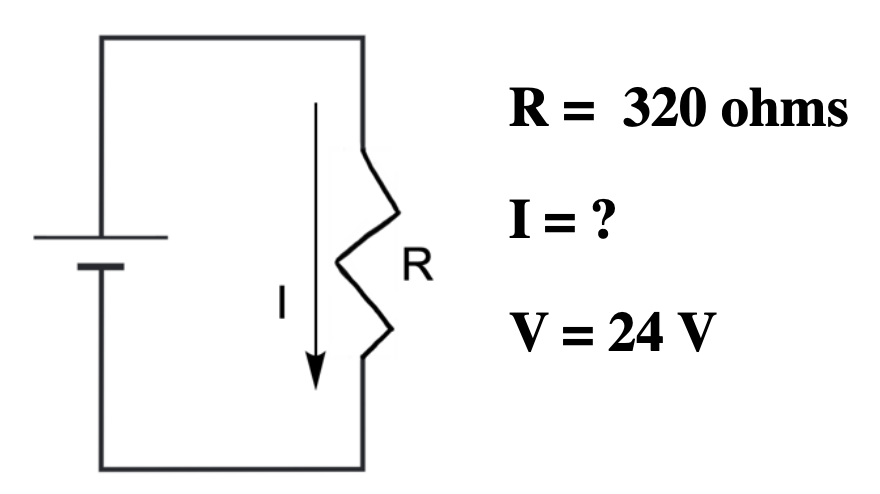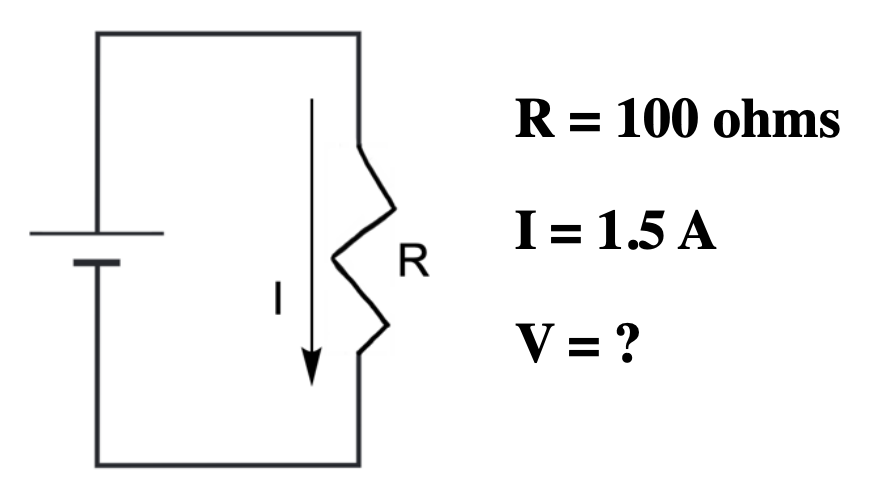Clutch Prep is now a part of Pearson
Ch.13 - Fundamentals of ElectrochemistryWorksheetSee all chapters

# Basic Concepts

See all sections
Sections
Basic Concepts
Electrochemical Cells
Standard Potentials
Nernst Equation
Standard Cell Potential & the Equilibrium Constant
###### Basic Redox Concepts

Concept #1: OXIDATION-REDUCTION (REDOX) reactions deal with the transfer of electrons from one reactant to another.

Concept #2: The movement of electrons from one electrode to another helps in the generation of voltage.

###### Basic Redox Concepts Calculations

Example #1:

Example #2: Basic Redoxs Calculations 1

Practice: Solve for the missing variable in the following circuit.Practice: Solve for the missing variable in the following circuit.Example #3: Basic Redoxs Calculations 2

Example #4: Basic Redoxs Calculations 2

Practice: A metal forms the salt MCl 3. Electrolysis of the molten salt with a current of 0.700 A for 6.63 h produced 3.00 g of the metal. What is the molar mass of the metal?

Example #5: Basic Redoxs Calculations 3

Example #6: Basic Redoxs Calculations 3

Example #7: Basic Redoxs Calculations 3

###### Balancing Redox Reactions

Concept #3: Generally, you will need to balance a redox reaction in an acidic or basic solution.

Example #8: Balance the following redox reaction in acidic solution.

Cr2O72– (aq) + H2O2 (aq)  → Cr3+ (aq) + H2O (l) + O2 (g)

Practice: Balance the following redox reaction in basic solution.

Cr2O72– (aq) + SO32– (aq)  →  Cr3+ (aq)  +  SO42– (aq)